# 微电网逆变器的改进型下垂控制策略An Improved Droop Control Strategy for Micro-Grid Inverter

• 全文下载: PDF(957KB)    PP.459-472   DOI: 10.12677/SG.2017.76051
• 下载量: 658  浏览量: 2,182   国家科技经费支持

In this paper, through the analysis of active and reactive power of traditional droop control algorithm, the voltage and current double loop control strategy is introduced. The virtual inductor causes the equivalent output impedance of the inverter into emotional, to provide a power equalization effect by adjusting the impedance matching degree; an improved droop control algorithm is proposed for multi-inverter parallel operation, and the influence of impedance on the circulation is weakened by the droop control parameters setting. The simulation results show that the improved droop control algorithm of multi-inverters parallel operation in micro-grid improves the performance of multi-inverters parallel operation. The circulating current problem of multi-inverters parallel operation is effectively reduced, and the reactive power balance effect of multi inverters parallel operation is greatly improved.

1. 引言

2. 传统下垂控制方法分析

U1∠θ1、U2∠θ2和U3∠θ3分别为分布式电源逆变器的空载输出电压；θ1、θ2、θ3为分布式电源逆变器的空载输出电压与母线电压的相角差。由图2可得逆变器i (i = 1, 2, 3)输出的有功功率和无功功率分别为

${P}_{i}=\frac{1}{|{Z}_{i}|}\left[\left(E{U}_{i}\mathrm{cos}{\theta }_{i}-{E}^{2}\right)\mathrm{cos}{\varnothing }_{i}+E{U}_{i}\mathrm{sin}{\theta }_{i}\mathrm{sin}{\varnothing }_{i}\right]$ (1)

${Q}_{i}=\frac{1}{|{Z}_{i}|}\left[\left(E{U}_{i}\mathrm{cos}{\theta }_{i}-{E}^{2}\right)\mathrm{sin}{\varnothing }_{i}-E{U}_{i}\mathrm{sin}{\theta }_{i}\mathrm{cos}{\varnothing }_{i}\right]$ (2)

$|{Z}_{i}|=\sqrt{{R}_{i}^{2}+{X}_{i}^{2}}$ (3)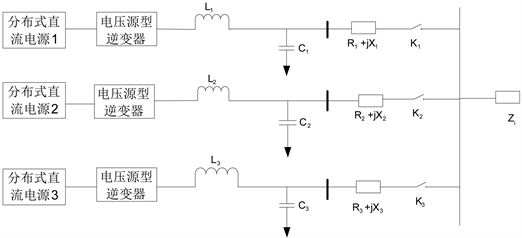Figure 1. A microgrid structure with 3 distributed sources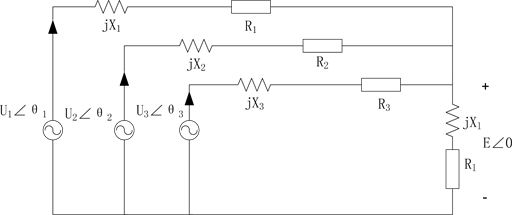Figure 2. Schematic diagram

${\varnothing }_{i}=\mathrm{arctan}\frac{{R}_{i}}{{X}_{i}}$ (4)

${P}_{i}=\frac{E{U}_{i}\mathrm{sin}{\theta }_{i}}{{X}_{i}}$ (5)

${Q}_{i}=\frac{{U}_{i}\left({U}_{i}-E\mathrm{cos}{\theta }_{i}\right)}{{X}_{i}}$ (6)

${P}_{i}=\frac{E{U}_{i}}{{X}_{i}}{\theta }_{i}$ (7)

${Q}_{i}\cong \frac{{U}_{i}\left({U}_{i}-E\right)}{{X}_{i}}$ (8)

$\left\{\begin{array}{l}{f}_{i}={f}_{0i}-{m}_{i}\left({P}_{i}-{P}_{0i}\right)\\ {U}_{i}={U}_{0i}-{n}_{i}\left({Q}_{i}-{Q}_{0i}\right)\end{array}$ (9)

${f}_{0i}$${U}_{0i}$ 分别为逆变器的额定频率、额定电压；

${m}_{i}$${n}_{i}$ 分别为逆变器的有功/频率(P/f)、无功/电压(Q/U)下垂控制系数；

${P}_{i}$${Q}_{i}$ 分别为逆变器的有功功率和无功功率；

${P}_{0i}$${Q}_{0i}$ 分别为逆变器的额定有功功率、额定无功功率。

${P}_{i}=\frac{{U}_{i}\left({U}_{i}-E\mathrm{cos}{\theta }_{i}\right)}{{R}_{i}}$ (10)

${Q}_{i}=-\frac{E{U}_{i}\mathrm{sin}{\theta }_{i}}{{R}_{i}}$ (11)

${P}_{i}=\frac{{U}_{i}\left({U}_{i}-E\right)}{{R}_{i}}$ (12)

${Q}_{i}\cong -\frac{E{U}_{i}{\theta }_{i}}{{R}_{i}}$ (13)

$\left\{\begin{array}{l}{f}_{i}={f}_{0i}-{u}_{i}\left({Q}_{i}-{Q}_{0i}\right)\\ {U}_{i}={U}_{0i}-{v}_{i}\left({P}_{i}-{P}_{0i}\right)\end{array}$ (14)

2.1. 传统下垂控制的功率分配

2.1.1. 无功功率分配

$\left\{\begin{array}{l}{f}_{i}={f}_{0i}-{u}_{i}\left({Q}_{i}-{Q}_{0i}\right)\\ {U}_{i}={U}_{0i}-{v}_{i}\left({P}_{i}-{P}_{0i}\right)\end{array}$ (15)

${f}_{01}={f}_{02}={f}_{03}$ (16)

${u}_{1}{Q}_{01}={u}_{2}{Q}_{02}={u}_{3}{Q}_{03}$ (17)

${u}_{1}{Q}_{1}={u}_{2}{Q}_{2}={u}_{3}{Q}_{3}$ (18)

${u}_{1}\frac{E{U}_{1}}{{R}_{1}}{\theta }_{1}={u}_{2}\frac{E{U}_{2}}{{R}_{2}}{\theta }_{2}={u}_{3}\frac{E{U}_{3}}{{R}_{3}}{\theta }_{3}$ (19)

${\theta }_{1}={\theta }_{2}={\theta }_{3}$${U}_{1}={U}_{2}={U}_{3}$ ，则有下式成立

$\frac{{u}_{1}}{{R}_{1}}=\frac{{u}_{2}}{{R}_{2}}=\frac{{u}_{3}}{{R}_{3}}$ (20)

2.1.2. 有功功率分配

$\left\{\begin{array}{l}{f}_{i}={f}_{0i}-{u}_{i}\left({Q}_{i}-{Q}_{0i}\right)\\ {U}_{i}={U}_{0i}-{v}_{i}\left({P}_{i}-{P}_{0i}\right)\end{array}$ (21)

${U}_{01}={U}_{02}={U}_{03}$ (22)

${v}_{1}{P}_{01}={v}_{2}{P}_{02}={v}_{3}{P}_{03}$ (23)

${v}_{1}{P}_{1}={v}_{2}{P}_{2}={v}_{3}{P}_{3}$ (24)

${U}_{1}={U}_{2}={U}_{3}$ (25)

$\Delta U={U}_{i+1}-{U}_{i}={v}_{i+1}{P}_{i+1}-{v}_{i}{P}_{i}$ (26)

${P}_{i}=\frac{E{U}_{0i}-{E}^{2}}{{R}_{i}+{v}_{i}E}$ (27)

$\Delta U=\frac{{U}_{0i}-E}{1+\frac{{R}_{i+1}}{E{v}_{i+1}}}-\frac{{U}_{0i}-E}{1+\frac{{R}_{i}}{E{v}_{i}}}$ (29)

$\frac{{R}_{1}}{{v}_{1}}=\frac{{R}_{2}}{{v}_{2}}=\frac{{R}_{3}}{{v}_{3}}$ (30)

$\left\{\begin{array}{l}{u}_{1}{Q}_{01}={u}_{2}{Q}_{02}={u}_{3}{Q}_{03}\\ {v}_{1}{P}_{01}={v}_{2}{P}_{02}={v}_{3}{P}_{03}\\ {f}_{01}={f}_{02}={f}_{03}\\ {U}_{01}={U}_{02}={U}_{03}\end{array}$ (31)

$\left\{\begin{array}{l}{U}_{1}={U}_{2}={U}_{3}\\ \frac{{R}_{1}}{{v}_{1}}=\frac{{R}_{2}}{{v}_{2}}=\frac{{R}_{3}}{{v}_{3}}\end{array}⇔\left\{\begin{array}{l}{\theta }_{1}={\theta }_{2}={\theta }_{3}\\ \frac{{R}_{1}}{{u}_{1}}=\frac{{R}_{2}}{{u}_{2}}=\frac{{R}_{3}}{{u}_{3}}\end{array}$ (32)

3. 逆变器的控制策略及输出阻抗设计

3.1. 虚拟阻抗法

${V}_{i}={U}_{i}-{I}_{i}{Z}_{i}$ (33)

3.2. 虚拟阻抗的电容电流反馈控制逆变器结构分析Figure 3. Parallel operation equivalent diagram of 3 inverters with virtual impedance introduced

${u}_{ref}={u}_{ref}^{*}$ (34)

$u=\frac{\left({k}_{Pv}S+{k}_{iv}\right){k}_{pi}{k}_{PWM}}{\Delta }{u}_{ref}-\frac{L{s}^{2}}{\Delta }{i}_{0}$ (35)

$\Delta =LC{s}^{3}+{k}_{U}{k}_{pi}{k}_{WPM}C{S}^{2}+\left(1+{k}_{l}{k}_{pi}{k}_{WPM}{k}_{pv}\right)s+{k}_{U}{k}_{iv}{k}_{pi}{k}_{PWM}$ (36)

$\Delta \approx \left(1+{k}_{U}{k}_{pi}{k}_{WPM}{k}_{pv}\right)s+{k}_{U}{k}_{iv}{k}_{pi}{k}_{PWM}$ (37)

$u\approx \frac{\left({k}_{Pv}S+{k}_{iv}\right){k}_{pi}{k}_{PWM}}{\left(1+{k}_{U}{k}_{pi}{k}_{WPM}{k}_{pv}\right)s+{k}_{U}{k}_{iv}{k}_{pi}{k}_{PWM}}{u}_{ref}-\frac{L{s}^{2}}{\left(1+{k}_{U}{k}_{pi}{k}_{WPM}{k}_{pv}\right)s+{k}_{U}{k}_{iv}{k}_{pi}{k}_{PWM}\Delta }{i}_{0}$ (38)

${Z}_{out}\approx \frac{L{s}^{2}}{\left(1+{k}_{U}{k}_{pi}{k}_{WPM}{k}_{pv}\right)s+{k}_{U}{k}_{iv}{k}_{pi}{k}_{PWM}}=\frac{L{s}^{2}}{s+{k}_{U}{k}_{pi}{k}_{WPM}\left({k}_{pv}s+{k}_{iv}\right)}$ (39)

${u}_{ref}^{*}={u}_{ref}+{Z}_{V}\left(s\right){i}_{0}$ (40)

${Z}_{out}^{*}\left(s\right)=\frac{\left({k}_{Pv}S+{k}_{iv}\right){k}_{pi}{k}_{PWM}}{\left(1+{k}_{U}{k}_{pi}{k}_{WPM}{k}_{pv}\right)s+{k}_{U}{k}_{iv}{k}_{pi}{k}_{PWM}}{Z}_{V}\left(s\right)+{Z}_{out}\left(s\right)$ (41)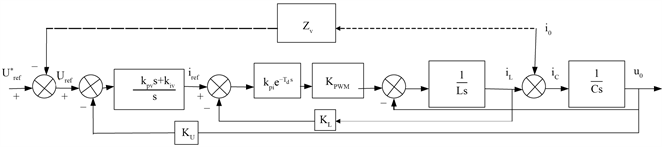Figure 4. Capacitor current feedback control structure of inverter with virtual impedance

4. 传统下垂控制算法的改进

$\left\{\begin{array}{l}{f}_{i}={f}_{0i}-{k}_{pf}{P}_{i}-{k}_{qf}{Q}_{i}\\ {U}_{i}={U}_{0i}-{k}_{pU}{P}_{i}-{k}_{qU}{Q}_{i}\end{array}$ (42)

${k}_{pf}=\frac{\Delta f}{{P}_{MAX}}$ (43)

${k}_{qU}=\frac{\Delta U}{{Q}_{MAX}}$ (44)

${P}_{i}=\frac{1}{|{Z}_{i}|}\left[\left(E{U}_{i}\mathrm{cos}{\theta }_{i}-{E}^{2}\right)\mathrm{cos}{\varnothing }_{i}+E{U}_{i}\mathrm{sin}{\theta }_{i}\mathrm{sin}{\varnothing }_{i}\right]$ (45)

$\frac{\partial {P}_{i}}{\partial {U}_{i}}=\frac{1}{|{Z}_{i}|}E\mathrm{cos}\left({\varnothing }_{i}-{\theta }_{i}\right)$ (46)

$\frac{\partial {P}_{i}}{\partial {\theta }_{i}}=\frac{1}{|{Z}_{i}|}E\mathrm{cos}\left({\varnothing }_{i}-{\theta }_{i}\right)$ (47)

$\frac{{K}_{pf}}{{K}_{pu}}=\frac{\partial {P}_{i}}{\partial {U}_{i}}/\frac{\partial {P}_{i}}{\partial P{\theta }_{i}}=\frac{1}{{U}_{i}}\frac{1}{\mathrm{tan}\left({\varnothing }_{i}-{\theta }_{i}\right)}$ (48)

${K}_{pU}={K}_{pf}{U}_{i}\mathrm{tan}\left({\varnothing }_{i}-{\theta }_{i}\right)\approx {K}_{pf}{U}_{i}\left(\mathrm{sin}{\varnothing }_{i}-\mathrm{sin}{\theta }_{i}\right)$ (49)

${K}_{pU}={K}_{pf}{U}_{i}\frac{{R}_{i}}{|{Z}_{i}|}$ (50)

${Q}_{i}=\frac{1}{|{Z}_{i}|}\left[\left(E{U}_{i}\mathrm{cos}{\theta }_{i}-{E}^{2}\right)\mathrm{sin}{\varnothing }_{i}-E{U}_{i}\mathrm{sin}{\theta }_{i}\mathrm{cos}{\varnothing }_{i}\right]$ (51)

$\frac{\partial {Q}_{i}}{\partial {U}_{i}}=\frac{1}{|{Z}_{i}|}E\mathrm{cos}\left({\varnothing }_{i}-{\theta }_{i}\right)$ (52)

$\frac{\partial {Q}_{i}}{\partial {\theta }_{i}}=\frac{1}{|{Z}_{i}|}E{U}_{i}\mathrm{cos}\left({\varnothing }_{i}-{\theta }_{i}\right)$ (53)

$\frac{{K}_{qf}}{{K}_{qU}}=\frac{\partial {Q}_{i}}{\partial {U}_{i}}/\frac{\partial {Q}_{i}}{\partial {\theta }_{i}}=-\frac{\mathrm{tan}\left({\varnothing }_{i}-{\theta }_{i}\right)}{{U}_{i}}$ (54)

${K}_{qf}=-{K}_{qU}\frac{\mathrm{tan}\left({\varnothing }_{i}-{\theta }_{i}\right)}{{U}_{i}}\approx -{K}_{qU}\frac{\mathrm{sin}{\varnothing }_{i}-\mathrm{sin}{\theta }_{i}}{{U}_{i}}$ (55)

${K}_{qf}\approx -\frac{{K}_{qU}}{{U}_{i}}\frac{{R}_{i}}{|{Z}_{i}|}$ (56)

$\left\{\begin{array}{l}{f}_{i}={f}_{0i}-{k}_{pf}{P}_{i}+\frac{{K}_{qU}}{{U}_{i}}\frac{{R}_{i}}{|{Z}_{i}|}{Q}_{i}\\ {U}_{i}={U}_{0i}-{K}_{pf}{U}_{i}\frac{{R}_{i}}{|{Z}_{i}|}{P}_{i}-{k}_{qU}{Q}_{i}\end{array}$ (57)

${R}_{i}=0$ 时，上式即为传统下垂控制算法，若引入感性虚拟阻抗，参考电压 ${u}_{ref}^{*}$ 则为

${u}_{ref}^{*}=\sqrt{2}{U}_{i}\mathrm{sin}\left(2\text{π}{f}_{i}t+{\theta }_{i}\right)$ (58)

5. 实验仿真验证Table 1. Simulation parameters of three inverters in parallel operation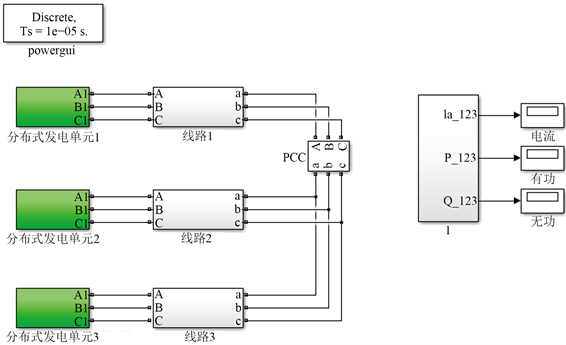Figure 5. Basic structure of 3 parallel inverters models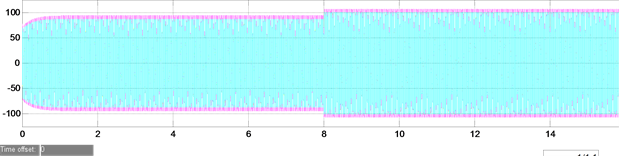(a)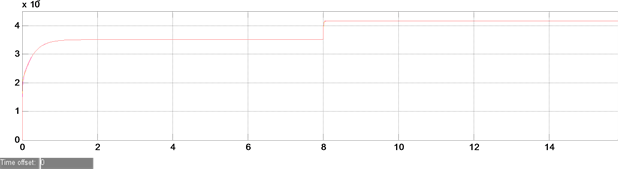(b)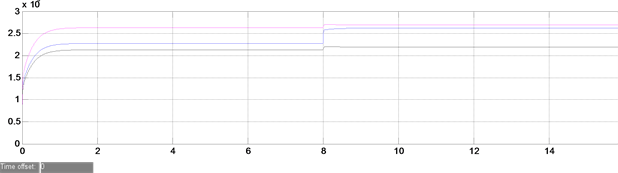(c)

Figure 6. Experimental simulation waveforms of traditional droop control algorithm without inductive virtual impedance. (a) Steady state current simulation results of 3 inverters in parallel operation; (b) Simulation results of output active power in parallel operation of 3 Inverters; (c) Simulation results of reactive power output in parallel operation of 3 inverters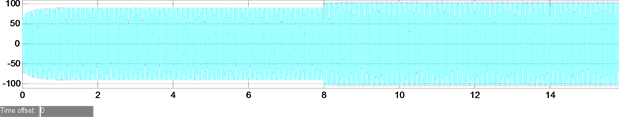(a)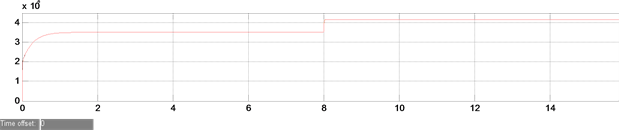(b)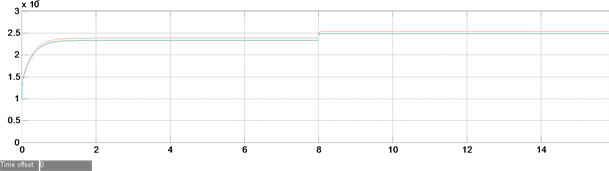(c)

Figure 7. Experimental waveforms of improved droop control algorithm with inductive virtual impedance. (a) Steady state current simulation results of 3 inverters in parallel operation; (b) Simulation results of output active power in parallel operation of 3 inverters; (c) Simulation results of reactive power output in parallel operation of 3 inverters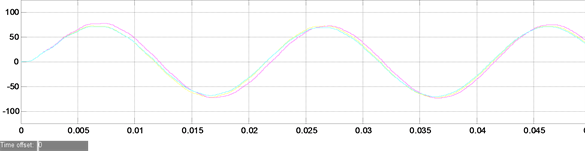(a)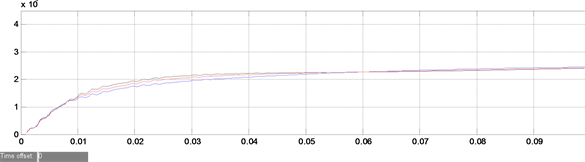(b)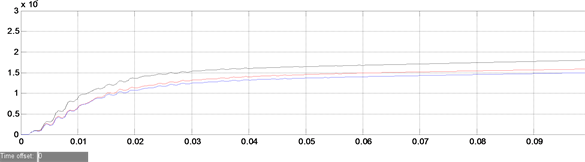(c)

Figure 8. The simulation waveform of traditional droop control algorithm is as follows. (a) Steady state current simulation results of 3 inverters in parallel operation; (b) Simulation results of output active power in parallel operation of 3 inverters; (c) Simulation results of reactive power output in parallel operation of 3 inverters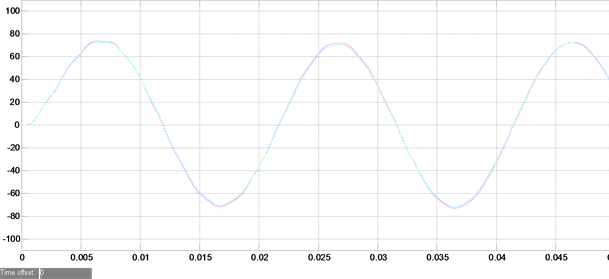(a)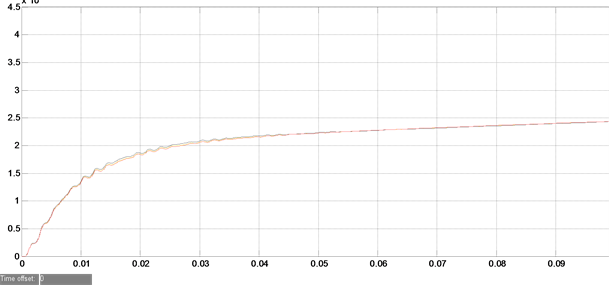(b)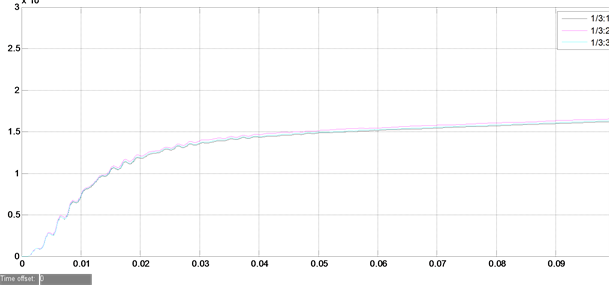(c)

Figure 9. Experimental simulation waveform chart of improved droop control algorithm. (a) Steady state current simulation results of 3 inverters in parallel operation; (b) Simulation results of output active power in parallel operation of 3 inverters; (c) Simulation results of reactive power output in parallel operation of 3 inverters

6. 结论

  黄宜平, 马晓轩. 微电网技术综述[J]. 电工技术学报, 2015(S1): 320-328.  李霞林, 郭力, 王成山, 李运帷. 直流微电网关键技术研究综述[J]. 中国电机工程学报, 2016, 36(1): 2-16.  杨新法, 苏建, 吕志鹏, 刘海涛, 李蕊. 微电网技术综述[J]. 中国电机工程学报, 2014, 34(1): 57-34.  吴玫蓉, 陶顺, 肖湘宁. 某海岛微电网的孤岛协调控制策略研究[J]. 电力系统, 2015, 34(9): 22-27.  马伟, 王维庆, 王海云. 微电网在孤岛模式运行时的下垂控制策略研究[J]. 电气传动, 2017, 47(1): 18-22.  徐晓龙. 微电网逆变器运行控制策略的研究[D]: [硕士学位论文]. 秦皇岛: 燕山大学, 2016.  杨琦, 马世英, 李胜, 唐晓骏, 李晓珺. 微型电网运行及控制设计[J]. 电工技术学报, 2011, 39(SI): 1-5.  李红萍, 杨洪耕, 曾巧燕. 孤岛型微电网中逆变器并联运行控制策略[J]. 电测与仪表, 2016, 53(6): 62-68.  鲍薇, 胡学浩, 李光辉, 鲍威宇. 独立型微电网中基于虚拟阻抗的改进下垂控制[J]. 电力系统保护与控制, 2013, 41(16): 7-13.  支娜, 张辉, 肖曦. 提高直流微电网动态特性的改进下垂控制策略研究[J]. 电工技术学报, 2016, 31(3): 31-38.  闫俊丽, 彭春华, 陈臣. 动态虚拟阻抗的低压微电网下垂控制策略[J]. 电力系统保护与控制, 2015, 43(21): 1-6.  郭诚, 顾军, 张武, 索亚楠, 喻景康, 罗心宇. 孤岛微网中基于虚拟负阻抗的改进下垂控制[J]. 电气传动, 2016, 46(6): 49-53.  张平, 石健将, 李荣贵, 龙江涛, 何湘宁. 低压微电网逆变器的“虚拟负阻抗”控制策略[J]. 中国电机工程学报, 2014, 34(12): 1844-1852.  天兵, 雷金勇, 许爱东, 郭小兵, 李鹏, 杨萍. 低压微电网孤岛模式下改进下垂控制策略[J]. 水电能源科学, 34(3): 204-208.  高春凤, 杨仁刚, 王江波, 周献飞. 基于虚拟频率的微电网下垂控制策略设计[J]. 电网技术, 2013, 37(12): 3331-3335.